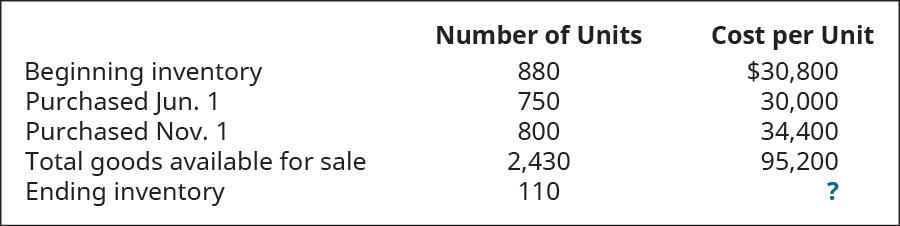# Bleistine Company had the following transactions for the month. Calculate the gross margin for the period for each of the following cost allocation methods, using periodic inventory updating. Assume that all units were sold for \$50 each. Provide your calculations. A. first-in, first-out (FIFO) B. last-in, first-out (LIFO) C. weighted average (AVG)FindFindarrow_forward

### Principles of Accounting Volume 1

19th Edition
OpenStax
Publisher: OpenStax College
ISBN: 9781947172685

#### Solutions

Chapter
SectionFindFindarrow_forward

### Principles of Accounting Volume 1

19th Edition
OpenStax
Publisher: OpenStax College
ISBN: 9781947172685
Chapter 10, Problem 6EB
Textbook Problem
63 views

## Bleistine Company had the following transactions for the month.Calculate the gross margin for the period for each of the following cost allocation methods, using periodic inventory updating. Assume that all units were sold for \$50 each. Provide your calculations.A. first-in, first-out (FIFO)B. last-in, first-out (LIFO)C. weighted average (AVG)

To determine

(a)

Concept introduction:

FIFO (First in first out): In FIFO method, the company issues first that material which is purchased earliest. This method provide highest gross margin in case of inflation.

LIFO (Last in first out): In LIFO method, the company issues first that material which comes in last in inventory. This method provide least gross margin in case of inflation.

Weighted average method: In this method, we issue material on random basis, so every type of goods has equal probability.

To calculate:

Gross Margin from FIFO method.

### Explanation of Solution

Gross margin as per FIFO= Sale price- (Total cost-(cost per unit* No of units remaini...

To determine

(b)

Concept introduction:

FIFO (First in first out): In FIFO method, the company issues first that material which is purchased earliest. This method provide highest gross margin in case of inflation.

LIFO (Last in first out): In LIFO method, the company issues first that material which comes in last in inventory. This method provide least gross margin in case of inflation.

Weighted average method: In this method, we issue material on random basis, so every type of goods has equal probability.

To calculate:

Gross Margin from LIFO method.

To determine

(c)

Concept introduction:

FIFO (First in first out): In FIFO method, the company issues first that material which is purchased earliest. This method provide highest gross margin in case of inflation.

LIFO (Last in first out): In LIFO method, the company issues first that material which comes in last in inventory. This method provide least gross margin in case of inflation.

Weighted average method: In this method, we issue material on random basis, so every type of goods has equal probability.

To calculate:

Gross Margin from weighted average method.

### Still sussing out bartleby?

Check out a sample textbook solution.

See a sample solution

#### The Solution to Your Study Problems

Bartleby provides explanations to thousands of textbook problems written by our experts, many with advanced degrees!

Get Started

Find more solutions based on key concepts
When are data in 3NF?

Accounting Information Systems

Name the two major types of financial statement analysis discussed in this chapter.

Managerial Accounting: The Cornerstone of Business Decision-Making

Changes in sales cause changes in profits. Would the profit change associated with sales changes be larger or s...

Fundamentals of Financial Management, Concise Edition (with Thomson ONE - Business School Edition, 1 term (6 months) Printed Access Card) (MindTap Course List)

What is the Laffer curve? What are the important implications of the Laffer curve?

Macroeconomics: Private and Public Choice (MindTap Course List)

What is the index of leading indicators? Why is it useful to macro policy-makers?

Economics: Private and Public Choice (MindTap Course List)